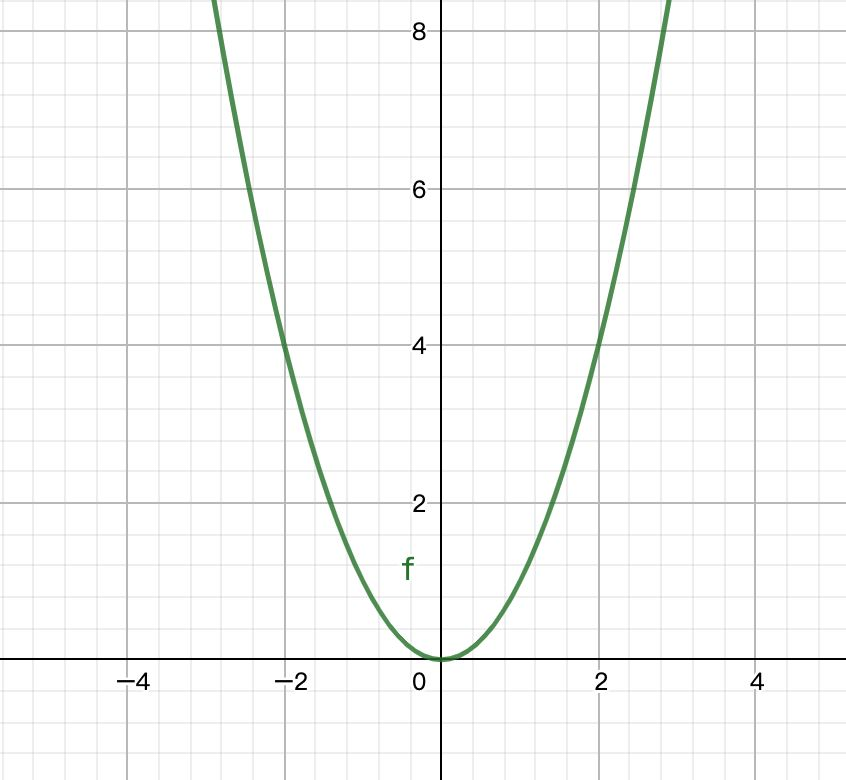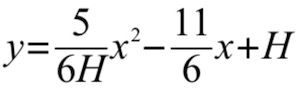# JS實現微信"炸屎"大作戰功能https://example.qiufeng.blue/wechat-feces/3-2-feces.html

Github地址：

https://github.com/hua1995116/node-demo/tree/master/wechat-feces

1.丟炸彈

2.炸彈爆炸

3.粑粑被炸開

4.所有人震動

## 具體實現

1.丟炸彈```var coords = { x: 300 };  // 起始點 為 x = 300
var tween = new TWEEN.Tween(coords)
.to({ x: 0  }, 1000) // 終點為 x = 0, 並且這個動作將在1秒內完成
.easing(TWEEN.Easing.Linear.None)	// 勻速```

```tween.onUpdate(function() {
var x = coords.x;
var y = 1/120 * x * x - 11/6 * x + 100;
box.style.setProperty('transform', 'translate(' + x + 'px, ' + y + 'px)');
})```

1.將方塊換成炸彈然，炸彈的形狀很簡單，我們可以通過 ps 將它從圖層中摳出來。

2.修改它運動時候的角度。2.炸彈爆炸

Lottie是一個庫，可以解析使用AE制作的動畫（需要用bodymovin導出為json格式），支持web、ios、android和react native。在web側，lottie-web庫可以解析導出的動畫json文件，並將其以svg或者canvas的方式將動畫繪制到我們頁面中。```<script src="https://cdn.bootcdn.net/ajax/libs/lottie-web/5.7.8/lottie.min.js"></script>
<body>
<div class="bodymovin"></div>
<script>
container: document.querySelector('.bodymovin'), // 要包含該動畫的dom元素
renderer: 'svg', // 渲染方式，svg、canvas、html（輕量版僅svg渲染）
loop: true, // 是否循環播放
autoplay: true, // 是否自動播放
path: './bomb.json', // 動畫json文件路徑
});
</script>```

```tween.onComplete(function () {
// 寫爆炸動畫
})```3.粑粑被炸開

3.1形狀```.feces {
position: absolute;
background-image: url(./feces.jpg);
background-size: 100%;
background-position: center;
background-repeat: no-repeat;
width: 80px;
height: 80px;
transform-origin: center;
}```
```// 創建一個粑粑元素
function createfeces(scale = 1) {
const fece = document.createElement('div');
fece.className = 'feces';
// 由於粑粑有大有小，有方向，因此預留瞭值。
const symbol = Math.random() > 0.5 ? 1 : -1;
fece.style.transform = `scale(\${scale * 1.1}) rotate(\${symbol * 20 * Math.random()}deg)`
return fece;
}```

3.2位置```// 計算要生成的多個粑粑的位置
// 傳入的參數num為要生成的粑粑的數量
function randomPosition(num) {
const radius = 80; // 圓半徑
const randomX = Math.random() * radius // 任取0到半徑中的任意一個x
const y = Math.round(Math.sqrt(radius * radius - randomX * randomX)); // 確定一個第一象限在圓上的點
const radian = Math.atan(y / randomX); // 這個點的弧度值

const step = Math.PI * 2 / num; // 每坨屎間距的弧度值

return new Array(num).fill(0).map((item, index) => {
const r = (index * step + radian)
// 將弧度為0 - 2 * PI
const tr = r > Math.PI * 2 ? r - Math.PI * 2 : r < 0 ? r + Math.PI * 2 : r;
return {
}
})

}``````randomPosition(6).map(item => ({ x: item.x + 150, y: item.y + 150 })) // 此處你也定義多於6個
``````const dis = Math.sqrt((end.x - 150) * (end.x - 150) + (end.y - 150) * (end.y - 150)); // 由於此時已經平移 150 ，因此需要計算距離中心點的距離
const r = Math.pow(2/3, dis / length); // 要縮放的比例``````function randomPosition(num) {
...
return new Array(num).fill(0).map((item, index) => {
const r = (index * step + radian)
const tr = r > Math.PI * 2 ? r - Math.PI * 2 : r < 0 ? r + Math.PI * 2 : r;
return {
// 增加隨機值
x: length * Math.sin(tr) + (Math.random() > 0.5 ? 1 : -1) * 10 * Math.random(),
y: length * Math.cos(tr) + (Math.random() > 0.5 ? 1 : -1) * 10 * Math.random(),
}
})
}```3.3角度

```function createfeces(scale) {
const fece = document.createElement('div');
fece.className = 'feces';
const symbol = Math.random() > 0.5 ? 1 : -1; // 生成 -20 ~ 20 之間的隨機角度
fece.style.transform = `scale(\${scale}) rotate(\${symbol * 20 * Math.random()}deg)`
fece.style.opacity = '0';
return fece;
}```3.4動畫

```// 一開始的出現時候的動畫，從爆炸口沖出來
function initFece(end) {
...
const start = { x: 0, y: 100, z: 0 }; // 爆炸口
const tween = new TWEEN.Tween(start)
.to({ ...end, z: 1 }, 100)
.easing(TWEEN.Easing.Linear.None)
.onUpdate(function () {
fece.style.setProperty('top', `\${start.y}px`);
fece.style.setProperty('left', `\${start.x}px`);
fece.style.setProperty('opacity', `\${start.z}`);
})
.onComplete(function () {
initDown(start, fece).start(); // 沖出完成，進行下落透明動畫
})
return tween;
}
// 下落同時變透明動畫
function initDown(start, fece) {
const s = {
y: start.y,
o: 1,
};
const e = { y: start.y + 80, o: 0 };
const tween = new TWEEN.Tween(s)
.to(e, 2000 + 500 * Math.random())
.onUpdate(function () {
fece.style.setProperty('top', `\${s.y}px`);
fece.style.setProperty('opacity', `\${s.o}`);
})
.onComplete(function () {
})
return tween;
}```3.5總結

• 先利用 `1 = x² + y²` 圓軌跡的特性，建立初步位置
• 再通過加入隨機值，使得整個分佈稍微不那麼規則一些
• 給粑粑添加隨機角度
• 讓中心粑粑更趨向於爆炸口
• 添加鏈式動畫出現和下落

4.所有人震動